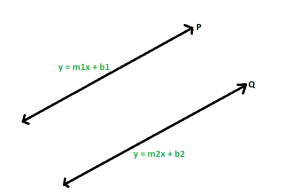Open in App
Not now

# Slope of the line parallel to the line with the given slope

• Last Updated : 08 Dec, 2022

Given an integer m which is the slope of a line, the task is to find the slope of the line which is parallel to the given line.

Examples:

Input: m = 2
Output: 2

Input: m = -3
Output: -3

Approach:Let P and Q be two parallel lines with equations y = m1x + b1, and y = m2x + b2 respectively. Here m1 and m2 are the slopes of the lines respectively. Now as the lines are parallel, they don’t have any intersecting point, and hence there will be no system of solutions for the lines. So, let us try to solve the equations,

For y, m1x + b1 = m2x + b2
m1x – m2x = b2 – b1
x(m1 – m2) = b2 – b1
The only way there can be no solution for x is for m1 – m2 to be equal to zero.
m1 – m2 = 0
This gives us m1 = m2 and the slopes are equal.

Below is the implementation of the above approach:

## C++

 `// C++ implementation of the approach` `#include ` `using` `namespace` `std;`   `// Function to return the slope` `// of the line which is parallel to` `// the line with the given slope` `double` `getSlope(``double` `m)` `{` `    ``return` `m;` `}`   `// Driver code` `int` `main()` `{` `    ``double` `m = 2;` `    ``cout << getSlope(m);`   `    ``return` `0;` `}`

## Java

 `// Java implementation of the approach` `import` `java.io.*;` `public` `class` `GfG {`   `    ``// Function to return the slope` `    ``// of the line which is parallel to` `    ``// the line with the given slope` `    ``static` `double` `getSlope(``double` `m) { ``return` `m; }`   `    ``// Driver code` `    ``public` `static` `void` `main(String[] args)` `    ``{` `        ``double` `m = ``2``;` `        ``System.out.println(getSlope(m));` `    ``}` `}`   `// This code is contributed by Code_Mech.`

## Python3

 `# Python3 implementation of the approach`   `# Function to return the slope` `# of the line which is parallel to` `# the line with the given slope` `def` `getSlope(m):`   `    ``return` `m;`   `# Driver code` `m ``=` `2``;` `print``(getSlope(m));`   `# This code is contributed` `# by Akanksha Rai`

## C#

 `// C# implementation of the approach` `class` `GFG` `{` `    `  `// Function to return the slope` `// of the line which is parallel to` `// the line with the given slope` `static` `double` `getSlope(``double` `m)` `{` `    ``return` `m;` `}`   `// Driver code` `static` `void` `Main()` `{` `    ``double` `m = 2;` `    ``System.Console.Write(getSlope(m));` `}` `}`   `// This code is contributed by mits`

## PHP

 ``

## Javascript

 ``

Output

`2`

Time Complexity: O(1)
Auxiliary Space: O(1)

My Personal Notes arrow_drop_up
Related Articles